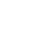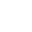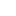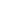# Prim’s Algorithm – Minimum Spanning Tree (MST)

What is Prim’s algorithm?

• Prim’s algorithm is a greedy algorithm.
• It finds a minimum spanning tree for a weighted undirected graph.
• This means it finds a subset of the edges that forms a tree that includes every vertex, where the total weight of all the edges in the tree is minimized.
• The algorithm operates by building this tree one vertex at a time, from an arbitrary starting vertex, at each step adding the cheapest possible connection from the tree to another vertex.

Example:How to implement Prim’s algorithm?

2. Maintain a set mst[] to keep track to vertices included in minimum spanning tree.
3. Assign a key value to all the vertices, (say key []) and initialize all the keys with +∞ (Infinity) except the first vertex. (We will start with this vertex, for which key will be 0).
4. Key value in step 3 will be used in making decision that which next vertex and edge will be included in the mst[]. We will pick the vertex which is not included in mst[] and has the minimum key. So at the beginning the first vertex will be picked first.
5. Repeat the following steps until all vertices are processed
1. Pick the vertex u which is not in mst[] and has minimum key.
2. Add vertex u to mst[].
3. Loop over all the adjacent vertices of u
• For adjacent vertex v, if v is not in mst[] and edge u-v weight is less than the key of vertex u, key[u] then update the key[u]= edge u-v weight.
4. Return mst[].

Please see the animation below for better understanding.Ways to Implement:

Time Complexity:

The time complexity of Prim’s algorithm depends on the data structures used for the graph and for ordering the edges by weight.

 Data Structure of Graph Ordering Time Complexity Adjacency Matrix Searching O(|V|2) Adjacency List Binary Heap O(|E|log|V|) Adjacency List Priority Queue with decrease key O(|E|2log|V|) Adjacency List Priority Queue without decrease key – Better Implementation O(|E|log|V|)

Reference – Wiki

__________________________________________________
Top Companies Interview Questions..-

If you find anything incorrect or you feel that there is any better approach to solve the above problem, please write comment.
__________________________________________________

#### You may also like...

This site uses Akismet to reduce spam. Learn how your comment data is processed.